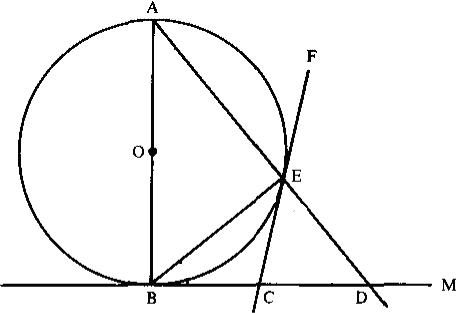$\def\bbN{{\mathbb N}} \def\bbQ{{\mathbb Q}} \def\bbR{{\mathbb R}} \def\bbZ{{\mathbb Z}}$
 © | Dror Bar-Natan: Classes: 2014-15: Math 475 - Problem Solving Seminar: (31) Next: Handout for February 26, "Divide into Cases", "Work Backwards", Pigeonhole Previous: Blackboards for Tuesday February 24

# Handout for February 24, "Divide into Cases" and "Work Backwards"

Reading. Sections 1.8 and 2.6 of Larson's textbook.

Next Quiz. Tuesday March 3, on these two sections, this handout, and maybe more, as will be announced in class.

Problem 1 (Larson's 2.5.11, modified).

1. Let $R_n$ denote the number of ways of placing $n$ nonattacking rooks on an $n\times n$ chessboard so that the resulting arrangement is symmetric about a $90^\circ$ clockwise rotation of the board about its centre. Compute $R_n$.
2. Let $S_n$ denote the number of ways of placing $n$ nonattacking rooks on an $n\times n$ chessboard so that the resulting arrangement is symmetric about the centre of the board. Compute $S_n$.
3. Let $T_n$ denote the number of ways of placing $n$ nonattacking rooks on an $n\times n$ chessboard so that the resulting arrangement is symmetric about both diagonals. Compute $T_n$.

Problem 2 (Larson's 2.5.13).

1. A derangement is a permutation $\sigma\in S_n$ such that for every $i$, $\sigma i\neq i$. Let $g_n$ be the number of derangements in $S_n$. Show that $g_1=0,\quad g_2=1,\quad g_n=(n-1)(g_{n-1}+g_{n-2}).$ Hint. A derangement interchanges $1$ with some other element, or not.
2. Let $f_n$ be the number of permutations in $S_n$ that have exactly one fixed point (namely, exactly one $i$ such that $\sigma i=i$). Show that $|f_n-g_n|=1$.Problem 3 (Larson's 1.8.1, modified).

1. Let $0<\alpha<\pi$. Show that $\frac{\sin\theta + \sin(\theta+\alpha)}{\cos\theta-\cos(\theta+\alpha)}$ is independent of $\theta$ for $0\leq\theta\leq\alpha$.
2. Can you find a geometric interpretation for this fact?

Problem 4 (Larson's 1.8.3). In the figure on the right, everything is as it seems: $O$ is the centre, $AB$ is a diameter, $CE$ and $BC$ are tangents, and all lines are straight. Show that $BC=CD$.